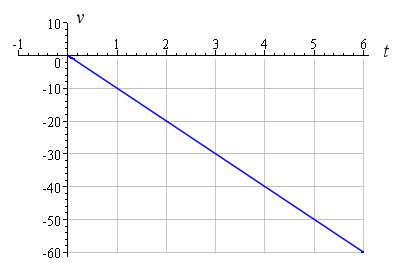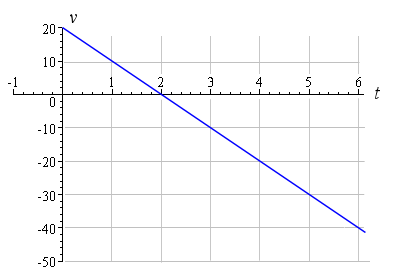Search IntMath
Close

# The IntMath Newsletter - 3 Jun 2008

By Murray Bourne, 03 Jun 2008

2. Math tips - (a) Slope-intercept form; (b) Are you future-proof?
3. From the math blog
4. Believe in yourself

## 1. Oops - repeated Newsletters

I'm really sorry that some of you received 2 or 3 copies of the 19 May Newsletter. I think I've finally squashed that coding bug now.

## 2. Math tips - (a) Slope-intercept form; (b) Are you future-proof?

a. Slope-intercept form

I drop a rock over a cliff. The speed of that rock at the beginning is 0 m/s. After 1 second, it is now traveling at almost -10 m/s (we usually regard "down" as "negative"). After 2 seconds, its velocity is around -20 m/s. Another second passes, and now the velocity is -30 m/s.

Each second, the rock's speed increases by around 10 m/s. We say that the relationship between time and velocity is linear, because if we plot time against velocity, we get a straight line graph. There is a linear relationship between the time and velocity.

In this case, the slope of our straight line graph is -10 (since the increase in velocity every second is -10 m/s) and the y-intercept (actually v-intercept, because we are talking about velocity on the vertical axis) is 0, since the rock starts at 0 m/s.

Here's the graph.Using slope intercept form (y = mx + b) for our graph, we write:

v = -10 t

In this case, the slope is given by m = -10 and the vertical intercept is b = 0.

[The actual acceleration due to gravity is 9.80665 m/s2, so after 3 seconds, the rock is traveling at 29.42 m/s.]

Now, let's start again and this time we'll throw the rock up at 20 m/s first. Gravity will act on it and slow it down, then the rock will drop into the valley. After 1 second, the rock is traveling (up) at +10 m/s. One second later it is moving at 0 m/s (it is at the top of its motion). After 3 seconds, it is going down at -10 m/s. Each second it will be going 10 m/s faster (in the downward direction).

Our linear graph will now start at positive 20 on the (vertical) velocity axis. It will have the same slope as the first graph (-10) since gravity is acting on it, but the intercept is different.Now, in y = mx + b form, our straight line is:

v = -10t + 20.

The slope is -10 (m/s2 - it's an acceleration) and the vertical intercept is 20 (the units are m/s for velocity).

The graphs only have positive values for t since negative values have no meaning in this application.

The concept of linear relationships has many applications in science (eg distance vs time if speed is constant) , economics (supply and demand - the cost of an item goes up if it is in demand and supply is short), biometrics (height vs weight of people), and many other fields.

See more on straight lines and slope-intercept form.

b. Are you future-proof?

Most people are expecting oil prices to remain high, and this will have a big impact on the way we learn and work in the future. Forget commuting, learning and working on-line is the future.

I wrote this math tip in its own post: Are you future proof?

Check it out. Do you agree with what I am suggesting? Will that be true in your country, do you think?

## 3. From the Math Blog

1) Friday Math Movie - Math Illuminated
Mathematics Illuminated is an award winning video series from Annenberg Media.

Some of the video topics include Primes, Infinity, Topology, Game Theory and Chaos.

2) Are you future proof?
How will high fuel prices affect the way we learn and work over the next 20 years or so?

There is a good chance that we will do more learning - and working - at home. And this will have a positive impact on the planet.

## 4. Believe in Yourself

You may have heard of the book (and DVD) called The Secret, by Rhonda Byrne, where she wrote about the Law of Attraction. This law basically says that we create our own success, depending on how we view our life. Think positive, and you are more likely to attract positive and encouraging people around you, and this feeds on itself. Think like a loser and you are sure to surround yourself with losers.

Do you sell yourself short? Do you believe what others have told you all your life, that you can't do stuff? You can change these self-destructive beliefs by creating what's called an affirmation, something like: "I have a goal of improving my grade in math next semester."

You then need to repeat your affirmation over and over and if you do this often enough, you'll change your brain's hard wiring.

Pretty soon you'll be thinking "success" and this will change your motivation for whatever you want − or need − to achieve. You'll start attracting other positive people and actually achieve something. So, time to get off your couch!

Here's to your success in math - or anything you do.

(With input from David Assaraf's related book, The Answer.)

See the 1 Comment below.

### Comment Preview

HTML: You can use simple tags like <b>, <a href="...">, etc.

To enter math, you can can either:

1. Use simple calculator-like input in the following format (surround your math in backticks, or qq on tablet or phone):
a^2 = sqrt(b^2 + c^2)
(See more on ASCIIMath syntax); or
2. Use simple LaTeX in the following format. Surround your math with $$ and $$.
$$\int g dx = \sqrt{\frac{a}{b}}$$
(This is standard simple LaTeX.)

NOTE: You can mix both types of math entry in your comment.